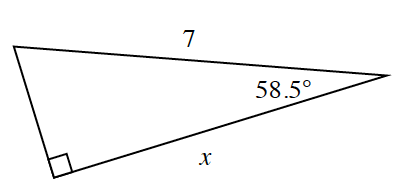### Home > CCG > Chapter 8 > Lesson 8.3.2 > Problem8-110

8-110.Krista is trying to solve for $x$ in the triangle at right.

1. What equation would Krista write?

With the given angle of $58.5°$, $x$ is the adjacent side and $7$ is the hypotenuse.
Which trigonometry ratio should you use?

2. Krista does not have a calculator, but she remembered something funny her friend Juanisha told her. Juanisha’s favorite sine ratio is sin $31.5°\approx0.522$ because 5/22 is Juanisha’s birthday! Without a calculator, use Juanisha’s favorite ratio to solve your equation from part (a).

Remember the sine of one acute angle in a right triangle equals the cosine of the other acute angle.
Find the measure of the missing angle in the triangle, then use sine ratio.